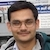# NAEST 2019 Screening Test: Bulbs Connected in SeriesBy

In screening round of National Anveshika Experimental Skills Test ( NAEST 2019 ), a question was asked on two bulbs of 100 W and 60 W. Similar problems were asked in NAEST 2014 and NAEST 2017.

## Question from NAEST 2019

A student bought a 100 Watt bulb and a 60 Watt bulb from the market. He connected them together such that both can be made on/off by a single switch. Choose the correct option(s) when the bulbs are switched on

1. The current passing through the 100 Watt bulb is less than the current through the 60 Watt bulbs.
2. The power consumed by the 100 Watt bulb is more than the power consumed by the 60 Watt bulb.
3. The current passing through both the bulbs is equal. Also, the power consumed by both bulbs is equal.
4. The voltage across the 100 Watt bulb is less than the voltage across the 60 Watt bulb.

Solution: The bulbs are usually connected in parallel as we seen in our houses. In a parallel connection, a 100 W bulb glows more than a 60 W bulb. In this video, 60 W bulb glows more than the 100 W bulb. These bulbs are connected in series as shown in the figure.In a series connection, the current through each bulb is the same. To find the current, we need to find the value of bulb resistances.

In India, bulb power is generally specified at a rated supply of $V=240$ Volt. Thus, the resistances of given bulbs of 100 W and 60 W powers are \begin{align} R_{100}&=\frac{V^2}{P_{100}}=\frac{240\times 240}{100}=576\,\Omega,\\ R_{60}&=\frac{V^2}{P_{60}}=\frac{240\times 240}{60}=960\,\Omega. \end{align}

The equivalent resistance of the series circuit is \begin{align} R_{eq}&=R_{100}+R_{60}\\ &=576+960=1536\,\Omega \end{align}Apply Ohm's law the get the current through the circuit \begin{align} i=\frac{V}{R_{eq}}=\frac{240}{1536}=0.16\,\mathrm{A} \end{align} The potential difference across two bulbs as \begin{align} V_{100}&=iR_{100}=0.16\times 576=90\,\mathrm{V}\\ V_{60}&=iR_{60}=0.16\times 960=150\,\mathrm{V} \end{align} The power consumed by two bulbs are \begin{align} P_{100}^c & =i^2 R_{100}=14\,\mathrm{W}, \\ P_{60}^c & =i^2 R_{60}=23\,\mathrm{W}. \end{align} Thus, the power consumed by 60 W bulb is more and hence it glows more.

Note: There is a small catch in the above analysis. We assumed that resistance of the bulb filament remains constant at its rated value. However, the temperature of the bulb filament varies with its temperature.

When two identical bulbs of rated power 100 W are connected in series than the power consumed by each bulb is only 25 W. The bulb glows with almost one-fourth of its normal intensity.

## Question from NAEST 2017

In NAEST 2017 question, two identical torch bulbs are connected across a 10 V battery in two different ways. The bulbs grow dimmer in case (i) and brighter in case (ii).

Which of the following is (are) correct in case (i)?

1. The bulbs are connected in series
2. The bulbs are connected in parallel
3. The voltage across each bulb is approximately 5 Volts
4. The voltage across each bulb is approximately 10 Volts
5. The current through two bulbs are equal
6. The current through two bulbs are not equal

Solution: The bulbs glow dimmer in series. The glow intensity is almost one-fourth of the normal intensity. The current through two bulbs are equal ($i/2$) and voltage across each bulb is 5 V.Which of the following is (are) correct in case (ii)?

1. The bulbs are connected in series
2. The bulbs are connected in parallel
3. The voltage across each bulb is approximately 5 Volts
4. The voltage across each bulb is approximately 10 Volts
5. The current through two bulbs are equal
6. The current through two bulbs are not equal

Solution: The bulbs glow brighter in parallel. The current through two bulbs are equal ($i$) and voltage across each bulb is 10 V.## Question from NAEST 2014

In first part of NAEST 2014 question, two bulbs of 25 W and 15 W are connected across a a power supply. Explain why intensity of two bulbs is reversed when connection is changed?

Initially, two bulbs of power rating $P_1=25$ W and $P_2=15$ W were connected in parallel to $V=250$ V power supply. In this case, the brightness of two bulbs was as expected i.e., $P_1^\text{parallel}=25$ W and $P_2^\text{parallel}=15$.

Then, these bulbs were connected in series to same supply. From power rating, the resistant of bulbs are \begin{align} R_1&=\frac{V^2}{P_1}=\frac{250\times250}{25}=2500\Omega,\\ R_2&=\frac{V^2}{P_2}=\frac{250\times250}{15}=4167\Omega, \end{align} (note that bulb with lower power rating has more resistance). In series, the current through both the bulbs is same and is equal to \begin{align} i_1&=i_2=\frac{V}{R_1+R_2}\\ &=\frac{250}{2500+4167}=0.037\,A. \end{align} The power output in series are \begin{align} P_1^\text{series}&=i_1^2R_1=0.037^2\times2500\\ &= 3.4\,W,\\ P_2^\text{series}&=i_2^2R_2=0.037^2\times4167\\ &= 5.7\,W. \end{align}

## Why Series Connection of Bulbs is Common in Rural India?

The series connection of the bulbs is quite popular in rural India. In villages, the voltage supply from the transformer fluctuates from its rated voltage of 240 V. If bulbs are connected in parallel they get fused whenever voltage goes high.

When supply voltage becomes large, the current through the bulb's filament increases proportionately. The large filament current produce excessive heat ($i^2 R t$) that results in a higher filament temperature. If filament temperature reaches the melting point then it got break (bulb gets fused).

To avoid this problem, villagers connect bulbs in series. The bulbs glow dimmer but are safe.

At many places, the power supply from the transformer is 440 V (phase to phase voltage). The bulbs are connected in series to keep the potential difference across each bulb within its rated supply voltage.